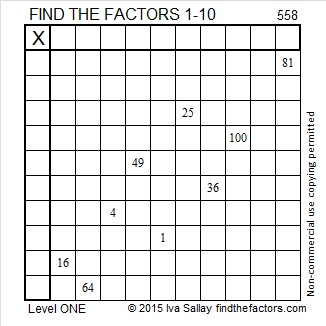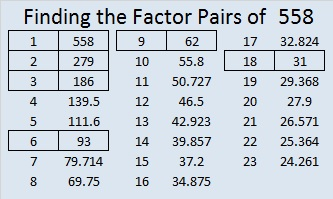# 558 and Level 1

558 is the sum of consecutive prime numbers 277 and 281.

558  is also the sum of all the prime numbers from 7 to 67.Print the puzzles or type the solution on this excel file: 10 Factors 2015-07-20

—————————————————————————————————

• 558 is a composite number.
• Prime factorization: 558 = 2 x 3 x 3 x 31, which can be written 558 = 2 x (3^2) x 31
• The exponents in the prime factorization are 1, 2, and 1. Adding one to each and multiplying we get (2 + 1)(1 + 1)(1 + 1) = 3 x 2 x 2 = 12. Therefore 558 has exactly 12 factors.
• Factors of 558: 1, 2, 3, 6, 9, 18, 31, 62, 93, 186, 279, 558
• Factor pairs: 558 = 1 x 558, 2 x 279, 3 x 186, 6 x 93, 9 x 62, or 18 x 31
• Taking the factor pair with the largest square number factor, we get √558 = (√9)(√62) = 3√62 ≈ 23.62202—————————————————————————————————